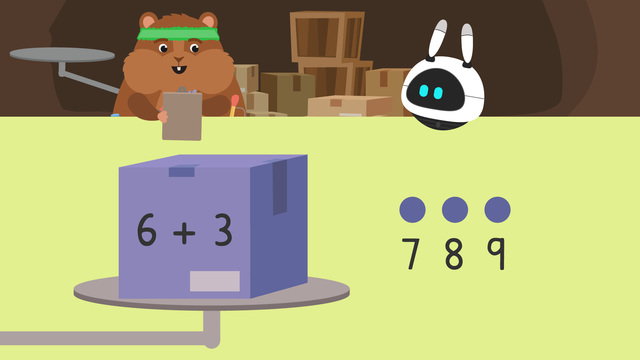# Equal / Not equalRating

Ø 3.6 / 5 ratings
The authorsTeam Digital
Equal / Not equal
CCSS.MATH.CONTENT.1.OA.D.7

## Basics on the topicEqual / Not equal

The equal sign can be found in any mathematical equation. It is important to understand what it means in order to be successful in mathematics. While you may be early on your mathematical journey, be assured that the equal sign will follow you through your learning all the time!

If you're looking for resources to help your child or student with understanding the equal sign, you've come to the right place! We offer a variety of tools and tips to make understanding of the equal sign easy. Please read our educational text about the equal sign, refer to the video and apply your knowledge by using our math worksheets.

## Equal or Not Equal? – Explanation

How can you know if two expressions are equal or not equal?

When two or more numbers, equations or calculations are equal, it means that they have the same value. When two or more numbers are not equal that means that they do not have the same value and one of them is either bigger than the other or smaller than the other.

We can use expressions and counting to help! When trying to figure out if two or more expressions are equal, follow the steps:

1. Start by solving the first expression

We either add two addends together to find the sum or complete the subtraction calculation to find the solution of the first expression.

2. Solve the second expression

Just like with the first equation, we either add two addends together to find the sum or complete the subtraction calculation to find the solution of the first expression.

You can do this using a variety of different strategies. You can use written or mental strategies to complete these mathematical equations.

Well done, you have reached the final step for figuring out if two calculations are equal or not. This step is very easy! If the sums are the same number, the expressions are equal! If not, they are not equal.

## Equal or Not Equal? – Guided Practice

Let’s practice finding if expressions are equal or not equal with the example below.Take a look at the expressions on these boxes. Do you think that they are equal or not equal? Remember to solve each expression and compare the answers to see if they are equal or not equal. The equations here are 5 + 4 and 6 + 3. Let’s solve them!

5 + 4 equals 9 and 6 + 3 equals 9 as well. These expressions are equal!Let’s try one more.Do you think that the expressions above are equal or not equal? Don’t forget to solve the expressions and compare the answers!

The sum of 6 + 2 is 8 and the sum of 7 + 3 is 10. So 6 + 2 does not equal 7 + 3. These expressions are not equal! How do you write the not equal symbol? The not equal symbol looks like . When you see the symbol , you need to say these numbers or equations do not equal, and by that you mean the sums are different.## Equal and Not Equal – Summary

When trying to figure out if expressions are equal to or not equal to, remembers to follow the steps:

Step # What to Do
1 Solve the first expression
2 Solve the second expression
3 Compare the sums! If they are the same number, it is equal!

Now you should be able to work out if sums and equations are equal or not equal. Feel free to watch the video explaining equal and not equal equations, or complete the worksheet on equal and not equal equations.